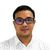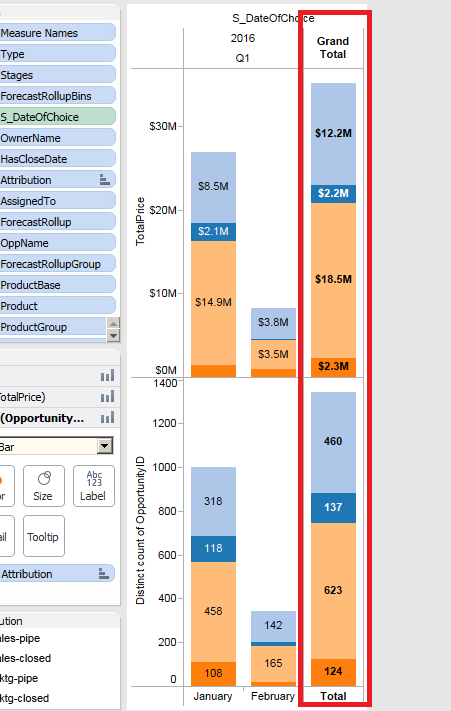# QlikView App Dev

Discussion Board for collaboration related to QlikView App Development.

cancel
Showing results for
Did you mean:Partner

## Hi guys, how can we create a chart (bar chart) with Grand total (please see the sample screenshot below)7 RepliesNot applicable

There is an option in Chart Property in Dimension Limit tab.

Chart Property->Dimension Limit tab->

Option Group Box

Check The Show Total Checkbox and label itPartner
Author

Thanks Nishit but, the new bar added is not segmented.MVP & Luminary

I think you need to add these TOTAL as an additionally dimension-value and respect this within your expression. This could be done with a calculated dimension like:

=valuelist(\$(=concat(distinct Month, ',')), 'Total')

and as expression someting like:

pick(match(valuelist(\$(=concat(distinct Month, ',')), 'Gesamt'), 1,2,3,4,5,6,7,8,9,10,11,12,'Gesamt'),
sum({< Monat = {1}>} [YourKPI]),
sum({< Month = {2}>} [YourKPI]),
sum({< Month = {3}>} [YourKPI]),
sum({< Month = {4}>} [YourKPI]),
sum({< Month = {5}>} [YourKPI]),
sum({< Month = {6}>} [YourKPI]),
sum({< Month = {7}>} [YourKPI]),
sum({< Month = {8}>} [YourKPI]),
sum({< Month = {9}>} [YourKPI]),
sum({< Month = {10}>} [YourKPI]),
sum({< Month = {11}>} [YourKPI]),
sum({< Month = {12}>} [YourKPI]),
sum({< Month = p(Month)>} [YourKPI]))

You might need some small adjustments to respect the year and/or a switch over a year or similar. But if add (per concatenate) these TOTAL within the script, maybe in an parallel field only for these purpose it would be easier to handle within the gui because you need only to query if your dimension-value is TOTAL or not.

- MarcusPartner
Author

thanks for the tips, but I'm using an cyclic group type of date. To drill down different dates.

how can I apply it to a cyclic type date

Thanks

DonMVP & Luminary

You could use the same approach like above with several calculated dimensions in a dimension group, like:

=valuelist(\$(=concat(distinct Date, ',')), 'Total') //Date

=valuelist(\$(=concat(distinct Week, ',')), 'Total') //Week

=valuelist(\$(=concat(distinct Month, ',')), 'Total') //Month

=valuelist(\$(=concat(distinct Year, ',')), 'Total') //Year

and the expression will be a bit longer and queried which dimension from the group is active:

pick(match(subfield(getcurrentfield(YourDimensionGroupName), '//', 2), 'Date', 'Week', 'Month', 'Year'),

..., // pick(match() expression like above only per Date or maybe on DAY

pick(match(valuelist(\$(=concat(distinct Month, ',')), 'Gesamt'), 1,2,3,4,5,6,7,8,9,10,11,12,'Gesamt'),
sum({< Monat = {1}>} [YourKPI]),
sum({< Month = {2}>} [YourKPI]),
sum({< Month = {3}>} [YourKPI]),
sum({< Month = {4}>} [YourKPI]),
sum({< Month = {5}>} [YourKPI]),
sum({< Month = {6}>} [YourKPI]),
sum({< Month = {7}>} [YourKPI]),
sum({< Month = {8}>} [YourKPI]),
sum({< Month = {9}>} [YourKPI]),
sum({< Month = {10}>} [YourKPI]),
sum({< Month = {11}>} [YourKPI]),
sum({< Month = {12}>} [YourKPI]),
sum({< Month = p(Month)>} [YourKPI])),

..., // pick(match() expression like above only per Week

...) // pick(match() expression like above only per Year

Not extremly nice but it should work. You should also consider to use several charts each specialized to a certain dimension or other things to keep the things simpler - maybe within a container and/or nested containers.

- MarcusPartner
Author

Thanks GuysIf not, please let us know with which part of this topic we can still help you.